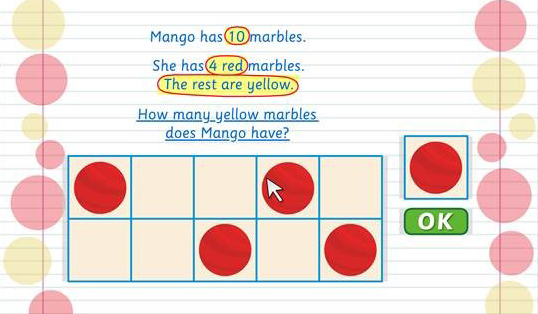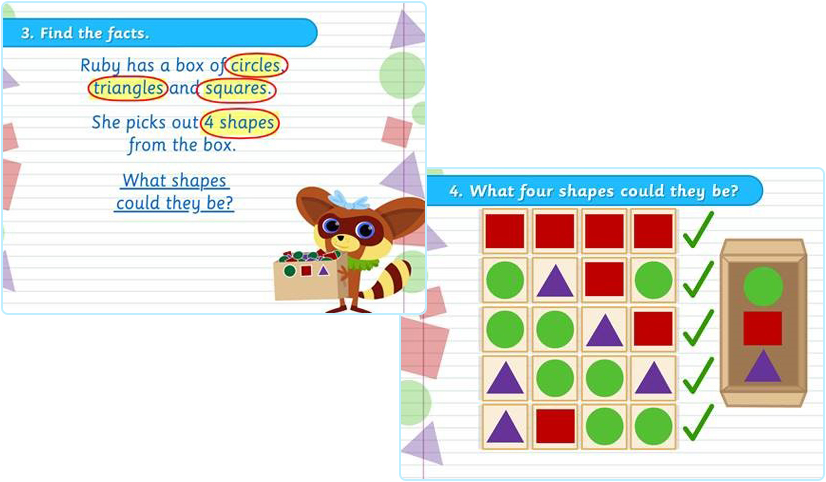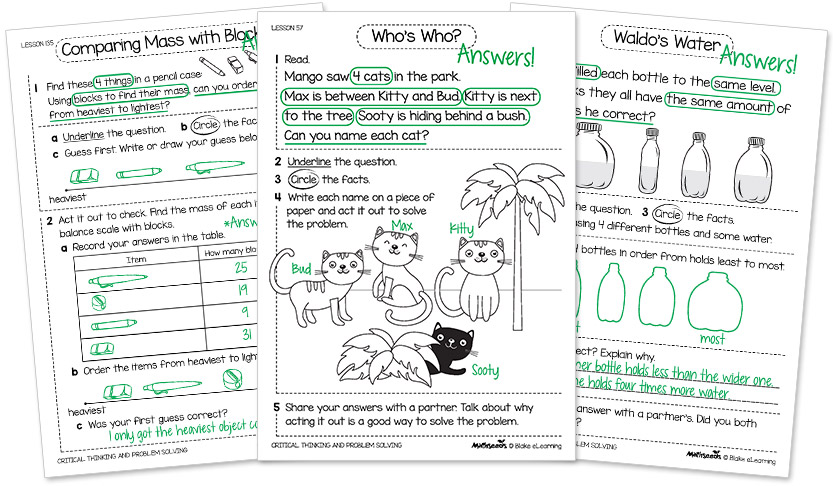## Start your free trial today!

1300 850 331 schools@mathseeds.com.au# ABC Mathseeds Problem Solving and Critical Thinking

### How does ABC Mathseeds develop higher‑level thinking skills?

The ABC Mathseeds lessons teach a variety of problem-solving strategies using interactive manipulatives to solve increasingly difficult math problems. Every ABC Mathseeds lesson also has a printable problem-solving task to target higher-level critical thinking skills. These challenge students from Kindergarten to Year 3 to develop a toolkit of strategies to reason, interpret, analyse and solve increasingly complex problems.1

## Online Lessons Teach StrategiesMathematical thinking and problem-solving in every lesson.

• Strategies, models and interactive manipulatives help students learn how to solve problems.
• Mathematical models include number lines, number charts, part-part whole, number patterns, place value, number bars, graphs and many more.
• Lessons promote thinking mathematically and developing number sense.
• Scaffolded learning enables students to apply their learning across a range of activities.
2

## A Structured Approach Builds Confident StudentsIdentify the question, find facts, apply and evaluate.

• Students tackle each problem using the same structure.
• Underline the question, circle the facts and choose a strategy.
• Allows students to develop critical thinking skills whatever their ability level.
3

## Printable Problem Solving Tasks for Every Lesson200 problem solving and critical thinking activities.

• Integrates problem solving into every lesson.
• Develops higher-level thinking skills across all mathematics domains.
• Strategies include act it out, draw diagrams, write equations, use tables, mental computation and logical thinking.
• Pages include collaborative elements and analysis where students are asked to think about their thinking, communicate this to others and justify their mathematical reasoning.
4

## Online GamesMini games in the Arcade prepare students for more difficult problem‑solving opportunities.

• Problem solving strategy games in the Games arcade develop logical thinking.
• Introduces early problem‑solving thinking.
• Fun, interactive and levelled to challenge students.
• Games include: Pack Your bags, Cow Jump, Where’s My shadow, Shape Tip, True or False.